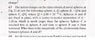# 3 Identical Charged Spheres

jegues

## Homework Statement

See figure attached from problem statement.

## The Attempt at a Solution

I've never really done a question of this type so I'm slow to get going.

I'm not sure what is happening when he touches the spheres with the others.

He first touches C to A:

They are postively charged so they should be in repulsion. Is there any exchange of charge or something happening here?

He then touches C to B:

C is postively charged while B is negatively charged so they should attract fairly well. Again, is there some sort of exchange in charge happening here when they touch?

What is happening when the spheres make contact!?

Once I understand this I will worry about trying to find the electrostatic force between spheres A and B.

Thanks again!

#### Attachments

•CH21-Q67.JPG
51 KB · Views: 383

Apphysicist
Since all the spheres are identical, when you touch C to A, the NET CHARGE of both spheres will equalize. Then the same when you touch C' to B (I say C' because it is not charged the same as C was originally)...it's a matter of you equally distributing those net charges to the spheres, so you know how much charge is on the spheres when you want to use coulomb's law.

jegues
Since all the spheres are identical, when you touch C to A, the NET CHARGE of both spheres will equalize. Then the same when you touch C' to B (I say C' because it is not charged the same as C was originally)...it's a matter of you equally distributing those net charges to the spheres, so you know how much charge is on the spheres when you want to use coulomb's law.

So I should find the charge of C' and A' then correct?

How do I find this?

I'm then going to have to find the charge of B' as well.

How do we do this?

Apphysicist
You're given all the initial charges of the spheres. Now, when you touch any two, they will equalize in terms of Net Charge...

e.g. I touch a +2mC sphere to a -2mC sphere...both sphere's come away ~neutrally charged.

jegues
You're given all the initial charges of the spheres. Now, when you touch any two, they will equalize in terms of Net Charge...

e.g. I touch a +2mC sphere to a -2mC sphere...both sphere's come away ~neutrally charged.

So if I sphere C (Q/2) to sphere A (Q) do they should both have a resulting charge of 3/4Q correct?

Then if I touch C'(3/4Q) to B they should have a resulting charge of 1/4Q.

Is this correct?